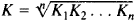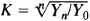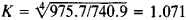# Rate of Growth

Also found in: Dictionary, Thesaurus, Medical, Financial, Acronyms.
The following article is from The Great Soviet Encyclopedia (1979). It might be outdated or ideologically biased.

## Rate of Growth

a relative statistical and planning indicator, characterizing the intensity of a phenomenon’s dynamics.

Rates of growth are obtained by dividing a phenomenon’s absolute level in a report or plan period by its absolute level in a base period, the period used for comparison (see alsoTIME SERIES). In base rates of growth, all the levels in a series are related to the level in a single period taken as the base period. In chain rates of growth, as distinct from base rates of growth, every level in a series is related to the level in the preceding period. Rates of growth are calculated as coefficients if the base level is taken as 1 and as percentages if the base level is taken as 100. The coefficients indicate the number of times by which the level in the report period exceeds the level in the base period, and the percentages indicate the relationship, expressed as a percentage, between the level in the report period and the level in the base period. The product of the chain rates of growth is equal to the base rate of growth. Rates of growth are used to compute rates of increase, which are equal to the rates of growth expressed in percentages minus 100 (see Table 1).

In Table 1, the base rates of growth reflect the intensity with which the production of electric power has grown over an entire time interval. The chain rates of growth show the intensity of growth by years. The rates of increase show the increase (in percentages) over the base year and each preceding year. Here, it is important to determine the amount represented by a 1 percent annual increase. As Table 1 shows, the amount represented by a 1 percent annual increase in the production of electric power has increased. In 1972 it was 8.03 billion kilowatt-hours (kW-hr) (57.0 divided by 7.1), and in 1974, 9.14 billion kW-hr (60.3 divided by 6.6). For characterizing the intensity of development by years, the differences in the base rates of increase (or growth) calculated in relation to a single initial level are of great importance. These growth points, as they are called, were 7.7 for 1972 (15.7 minus 8.0), 7.8 for 1973 (23.5 minus 15.7), and 8.2 for 1974 (31.7 minus 23.5). The high and stable rates of growth and rates of increase shown by the principal absolute indicators of the national

Table 1. Production of electric power in the USSR
19701971197219731974
Total production (billion kW-hr) ..............740.9800.4857.4914.7975.7
Rates of growth
Basocoefficients ..............11.0801.1571.2351.317
Base percentages ..............100108.0115.7123.5131.7
Chain coefficients ..............11.0801.0711.0671.066
Chain percentages ..............100108.0107.1106.7106.6
Rates of increase
Base rates ..............8.015.723.531.7
Chain rates ..............8.07.16.76.6

economy of the USSR demonstrate the economic advantages of the socialist economy over the capitalist economy.

The average annual rates of growth (and increase) provide a general picture of the intensity of economic development over several years. These rates are computed as the geometric mean of the annual rates according to the formulawhere K1, K2,...., Kn are the annual growth rates expressed as coefficients and n is the number of years. They are also computed according to the formulawhere Yn is the absolute level of the time series in the year n, Y0 is the absolute level of the time series in the base year, and n is the number of years (levels in a time series) in the time interval under investigation (not including the base year).

The average annual rate of growth for the production of electric power over the four years from 1971 to 1974 isor 107.1 percent, and the average annual rate of increase is 7.1 percent. The value of the average rate depends on the ratio between the initial level and the final level. Hence, the periods for which average rates are derived must be well chosen from an economic standpoint. As a rule, these periods should have a single direction of development and should be qualitatively uniform in this regard.

### REFERENCES

Kharlamov, A. I. Statisticheskie pokazateli tempov ekonomicheskogo razvitiia. Moscow, 1962.
Riauzov, N. N. Obshchaia teoriia statistiki, 2nd ed. Moscow, 1971.
Teoriia statistiki, 3rd ed. Moscow, 1975.

N. N. RIAUZOV

## growth rate

Rate of wood growth expressed as the number of annual rings per inch measured from pith to bark; sometimes used to rate soft-woods for strength.
McGraw-Hill Dictionary of Architecture and Construction. Copyright © 2003 by McGraw-Hill Companies, Inc.
References in periodicals archive ?
In the acute care sector, the rate of growth in spending on physician services increased to 4.2 percent, from 3.2 percent, but the rate of growth in spending on hospital care fell to 4.3 percent, from 4.9 percent.
In order to examine whether there is any acceleration/deceleration/constant in the growth of area/production/yield of selected agricultural crops in India, the following log quadratic form of equation (second degree polynomial equation) has been used for estimating the change in the rate of growth of area, production and yield of selected agricultural crops over time directly and it has been fitted to the time series points for the period 1970-71 to 2009-10.
The report said: "This rate of growth means that travel and tourism industry is expected to directly contribute pounds 35.6bn and almost 950,000 jobs to the British economy.
* Retail prescription drug spending (10% of total health care spending) grew only 1.2% to \$259.1 billion in 2010, a substantial slowdown from 5.1% growth in 2009, and the slowest rate of growth for prescription drug spending recorded in the NHE.
The rate of growth is the highest since April 2006 when PMI was at 56 percent, said Norbert J.
rate of growth of labor productivity until the firm begins to add
Summary: The stellar rate of growth at online fashion retailer ASOS appears to be slackening off despite virtually doubling annual profits.
Yesterday's figures from the Department for Communities and Local Government (CLG) showed the annual rate of growth slowed to 0.6% in June, down sharply from the 3% seen in the year to May.
In 2006, the rate of growth of private consumption declined to 3.0 percent.
Rodriguez acknowledged in an AP interview that the government's method of counting output "has an influence" on the high rate of growth. Cuba includes social services not counted in UN-standard measures of economic output.
At the company's current rate of growth and exploration, Makuch says the company expects to be able to boost those numbers to 24 million pounds of nickel and 100 million pounds of copper per year by 2010.
Based on statistics published by the International Monetary Fund (IMF) in September 2006, the rate of growth of Ghana's economy from 1995 through 2002 is calculated at an average of 4.3 percent per year.

Site: Follow: Share:
Open / Close